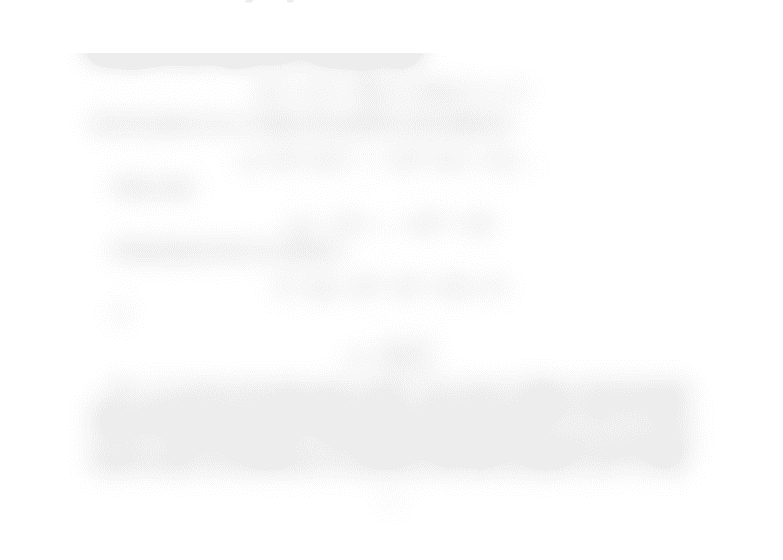# ECON 211 Chapter 3,4: Chapter #3,4 Notes

169 views2 pages
School
Department
Course
ProfessorEconomics 211
Some notes on chapters 3 and 4
The text deﬁnes a sequence as a function whose domain is the natural numbers {1,2,3, . . .}
(page 62). So if the range is <nwe could write the sequence as a countable subset of <n.
{a1, a2, a3, . . .}≡{ai}
i=1 ⊂ <n.
The limit of {ai}
i=1 as i→ ∞ exists and is a∈ <nif for each  > 0 there is some K
(which depends on ) in the natural numbers such that
{ai}
i=KN(a).
For example, {21
i,3 + 1
i}
i=1 is a sequence in <2whose limit is (2,3).
Aseries, say {si}
i=1, is a sequence that is based another sequence, say {ai}
i=1 ⊂ < (not
<n, n 2 ), with the structure that s1=a1,s2=a1+a2,s3=a1+a2+a3, and so on. Will
the limit of {si}
i=1 exist? If ai>0 for each iand
for i= 1,2, . . . ai+1
ai
rwhere 0 < r < 1,
then the answer is yes. Consider the geometric series deﬁned by
gi=Ar0+Ar1+. . . +Ari1for i= 1,2,....
Oberve that
rgi=Ar1+. . . +Ari1+Ari
Subtracting the last two equations
(1 r)gi=Ar0Ari=A1ri
or
gi=A1ri
1r
If r < 1rigoes to zero and gigoes to A/(1 r) as igoes to inﬁnity. So the geometric
series converges. If we can show this geometric series acts as an upper bound on {si}
i=1
under the conditions stated above then simust converge. Note that s1=a1,s2=a1+a2
a1+ra1=a1(1 + r), s3a1(1 + r+r2), and so on. Thus {si}
i=1 converges to some positive
number because it is bounded above by a geometric series that converges to a1/(1r)∈ <+.
1
Unlock document

This preview shows half of the first page of the document.
Unlock all 2 pages and 3 million more documents.

Already have an account? Log in

# Get access

Grade+
\$10 USD/m
Billed \$120 USD annually
Homework Help
Class Notes
Textbook Notes
40 Verified Answers
Study Guides
1 Booster Class
Class+
\$8 USD/m
Billed \$96 USD annually
Homework Help
Class Notes
Textbook Notes
30 Verified Answers
Study Guides
1 Booster Class